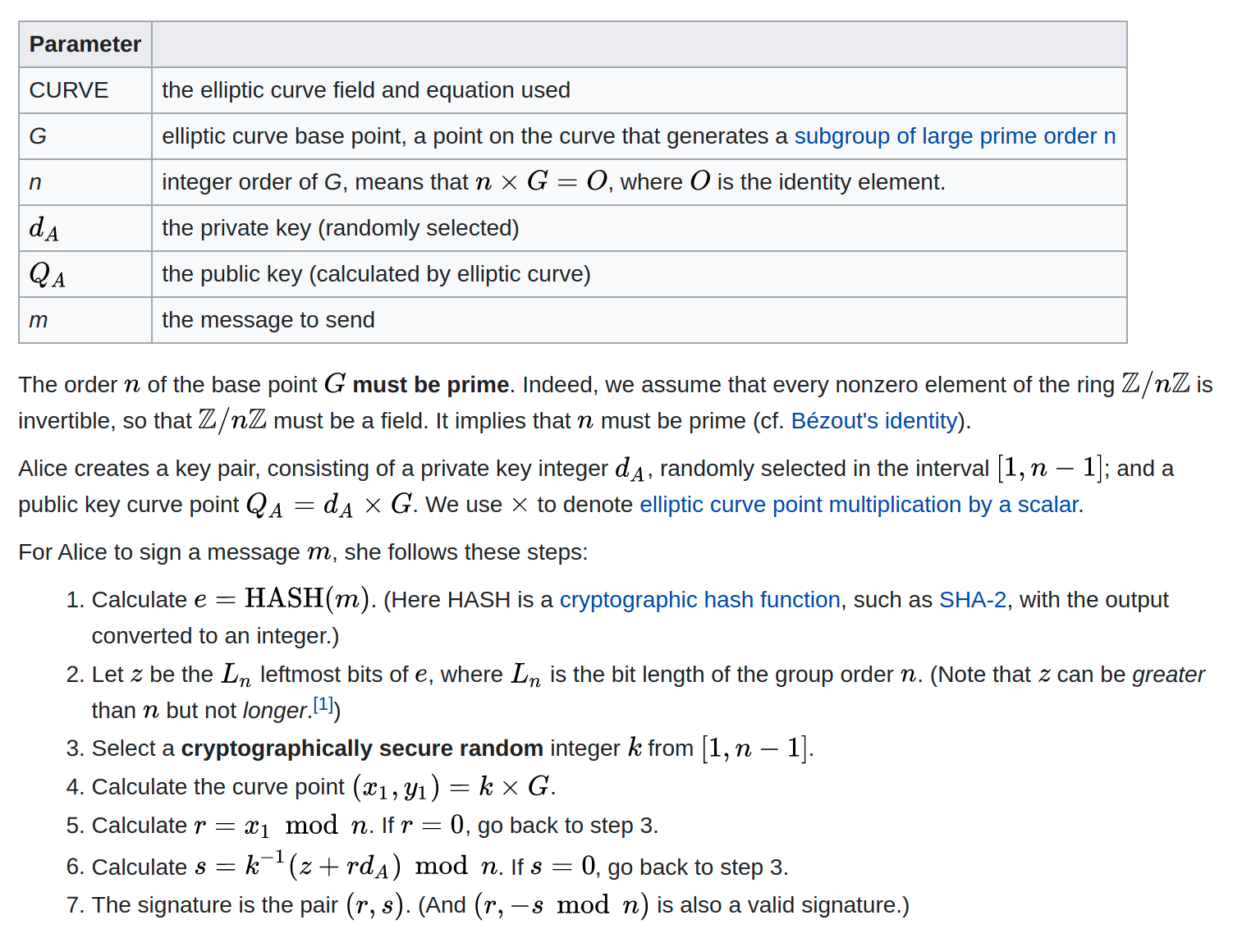### Modular Multiplicative Inverse (Clojure) in ECDSA

#### January 22, 2021    CryptographyClojure

I was putting together a Clojure library for RFC 6979’s deterministic elliptic curve digital signature algorithm (ECDSA)  on top of the JVM’s cryptographic primitives, and decided to try writing out ECDSA from scratch — separately from the production library! obviously — just to better understand what was going on with the deterministic k parameter that I was generating.

I got to step 6 of the algorithm described on the Wikipedia page before running out of vocabulary to Google the math that I needed to implement.It is a modular multiplicative inverse. If it were `s = k^-1 mod n` it would be straightforward enough to Google ‘mod inverse’, but there’s an extra step.

``````s = k^-1 * x (mod n)
= (k mod^-1 n) * x (mod n)
``````

It’s actually clearer in code I think.

``````(defn modular-multiplicative-inverse
"Find x in [0, p) such that (m * x) % p = n"
[n m p]
(let [n (biginteger n)
m (biginteger m)
p (biginteger p)]
(-> (.modInverse m p)
(.multiply n)
(.mod p))))
``````